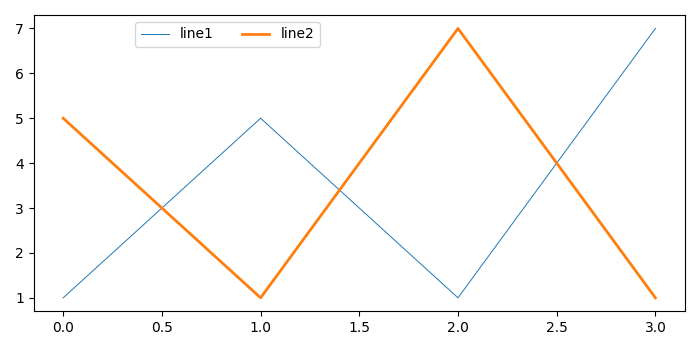# How to position and align a Matplotlib figure legend?

To position and align a matplotlib figure legend, we can take the following steps−

• Plot line1 and line2 using plot() method.
• Place a legend on the figure. Use bbox_to_anchor to set the position and make horizontal alignment of the legend elements.
• To display the figure, use show() method.

## Example

from matplotlib import pyplot as plt
plt.rcParams["figure.figsize"] = [7.00, 3.50]
plt.rcParams["figure.autolayout"] = True
line1, = plt.plot([1, 5, 1, 7], linewidth=0.7)
line2, = plt.plot([5, 1, 7, 1], linewidth=2.0)
plt.legend([line1, line2], ["line1", "line2"], bbox_to_anchor=(0.45, 1.0), ncol=2)
plt.show()

## Output